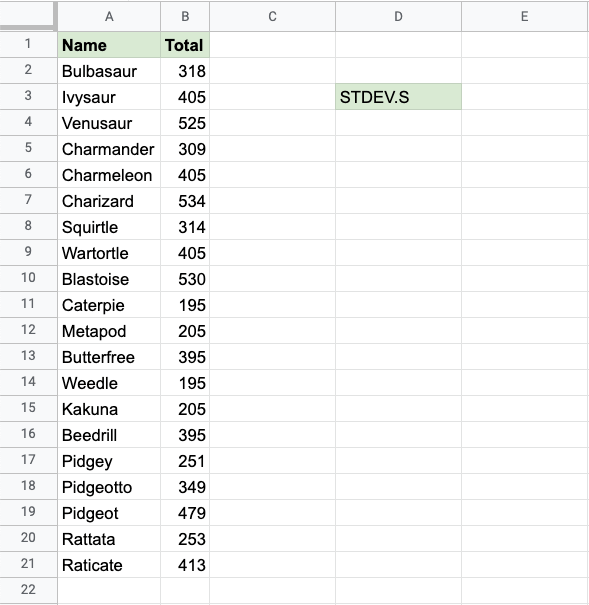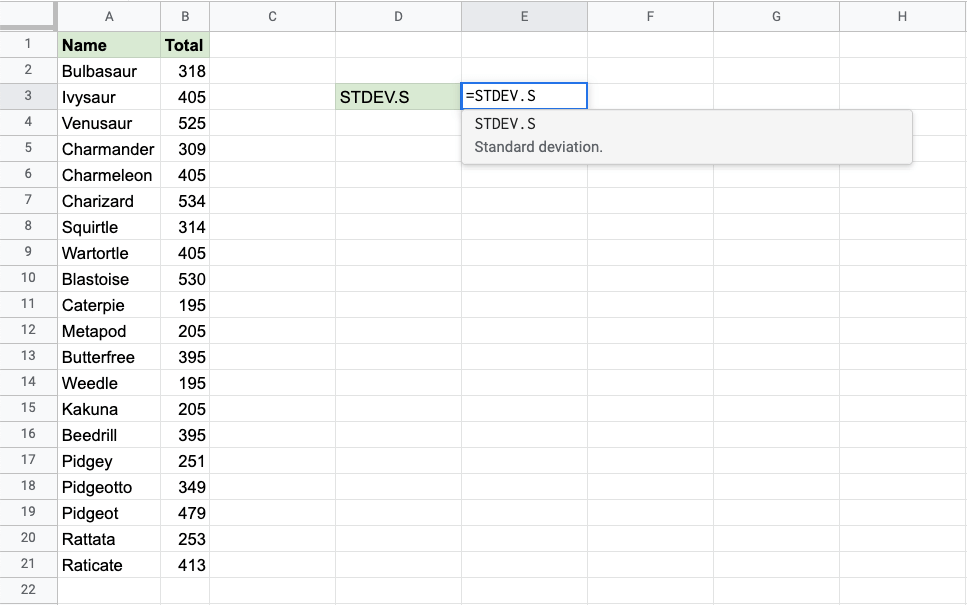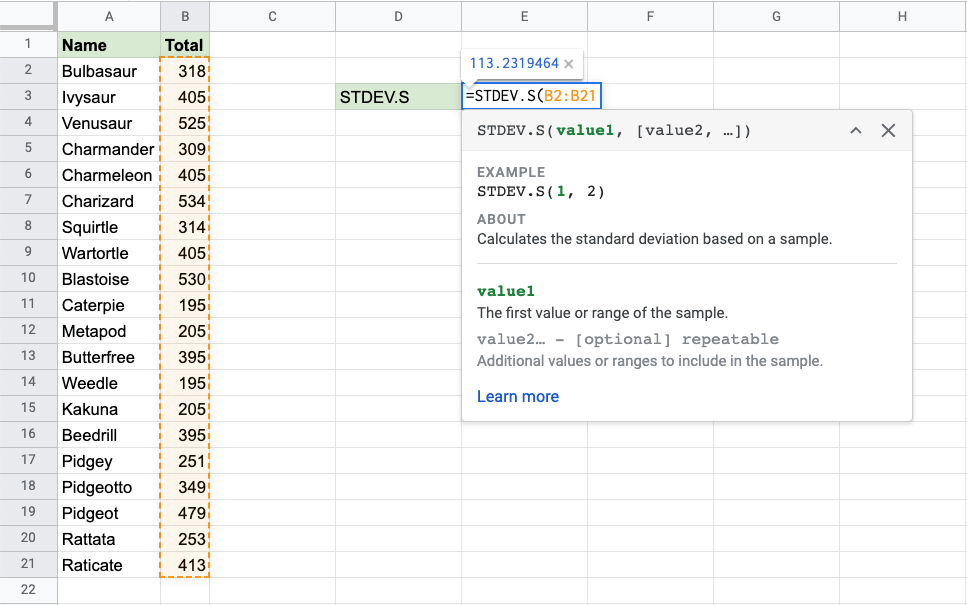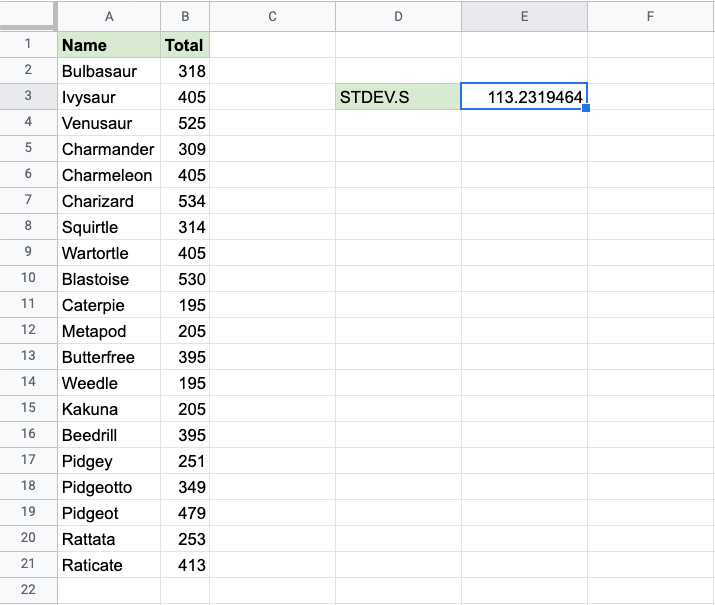## STDEV.S Function

The STDEV.S function is a premade function in Google Sheets, which calculates the Standard Deviation (Stdev) for a sample of a population.

It is typed `=STDEV.S` and gets a list of cells:

=STDEV.S(value1, [value2, ...])

The STDEV.S function ignores cells that do not contain numbers.

Note: Standard deviation (σ) measures how far a 'typical' observation is from the average of the data (μ). You can read more about standard deviation in the Statistics - Standard Deviation Chapter.

Tip: There is another function called `=STDEV.P` that can be used if you have the data for the entire population.

Let's have a look at an example!

## STDEV.S Function Example

Find the standard deviation of the total stats for the Pokemon in this sample:Copy Values

The STDEV.S function, step by step:

1. Select the cell `E3`
2. Type `=STDEV.S`
3. Click the STDEV.S command1. Specify the range `B2:B21` for the Total stats for the sample Pokemon
2. Hit enterNow, the function returns the standard deviation of all the Total stat values:The STDEV.S function has successfully returned the Standard Deviation for the sample as `113,2319464`.

W3Schools is optimized for learning and training. Examples might be simplified to improve reading and learning. Tutorials, references, and examples are constantly reviewed to avoid errors, but we cannot warrant full correctness of all content. While using W3Schools, you agree to have read and accepted our terms of use, cookie and privacy policy.